# TS Grewal Solution Class 12 Chapter 3 Goodwill Nature and Valuation

Read TS Grewal Accountancy Class 12 Solution Chapter 3 Goodwill Nature and Valuation 2022-23. Students should study TS Grewal Solutions Class 12 Accountancy available on Studiestoday.com with solved questions and answers. These chapter-wise answers for class 12 Accountancy have been prepared by the teacher of Grade 12. These TS Grewal class 12 Solutions have been designed as per the latest accountancy syllabus for class 12 and if practiced thoroughly can help you to score good marks in standard 12 Accounts class tests and examinations.

## Class 12 Accounts Chapter 3 Goodwill Nature and Valuation TS Grewal Solutions

TS Grewal Solutions for Chapter 3 Goodwill Nature and Valuation Class 12 Accounts have been provided below based on the latest TS Grewal Class 12 book. The answers have been prepared based on the latest 2022-2023 book for the current academic year. TS Grewal Solutions Class 12 will help students to improve their concepts and easily solve accountancy questions for class 12. Class 12 Grewal solutions should be revised regularly as more practice will help you get a better rank and easily solve more questions.

### Chapter 3 Goodwill Nature and Valuation TS Grewal Class 12 Solutions

TS Grewal Class 12 Chapter 3 provides a detailed understanding relating to the concepts of Goodwill and different methods used to value goodwill. As valuing goodwill is crucial, especially during mergers and acquisitions, as it can impact the valuation of the entire business. The valuation of goodwill is an important part of any business. Though its an intangible asset but its valuation is very essential for all types of businesses. Correct accounting treatment is essential to make sure the books of account show correct amount of goodwill. Its important to understand that goodwill is an essential element of a company's value, and understanding its nature and valuation is important for students as its used by all businesses and accountants. As valuing goodwill is a complex process, therefore detailed explanation has been provided in TS Grewal's Class 12 Accountancy book so that students can understand how to calculate it and easily solve questions in exams. Our Class 12 accountancy teachers have provided detailed solutions to all questions given in this chapter.

Solutions for T.S. Grewal's Double Entry Book Keeping:
Accounting for Not for Profit Organizations and Partnership Firms (Vol.1)
Textbook for CBSE Class 12
TS Grewal Solutions Class 12 Accountancy
Chapter 3
Goodwill: Nature and Valuation

Question 1. Define Goodwill.

Goodwill is a thing very easy to describe, very difficult to define. It is the benefit and advantage of the good name, reputation and connections of a business. It is the attractive force which brings in customers. It is one thing which distinguishes an old established business from a new business at its first start.

Question 2. State any three circumstances other than (i) admission of a new partner, (ii) retirement of a partner and (iii) death of a partner, when need for valuation of goodwill of a firm may arise.

The need for valuation of goodwill arises in the following circumstances:

1.)   When there is a change in the profit sharing ratio.

2.)   When partnership firm is sold as a going concern.

3.)   When two or more firms amalgamate.

Question 3. Give any two features of Goodwill.

Below are the features of Goodwill:-

1.)   It is an intangible asset i.e. an asset which cannot be seen and touched.

2.)   It does not have an existence separate from that of an enterprise. Thus, it has realisable value when business is sold.

Question 4. What is meant by Purchased Goodwill?

Purchased Goodwill is that goodwill which is acquired by a firm for a consideration, whether paid in cash of kind. For example, when a business is purchased and purchase consideration is more than value of net assets (i.e. Assets – Liabilities), the difference amount is the value of purchased goodwill.

Question 5. What is meant by Self-generated Goodwill?

Self-generated goodwill is that goodwill which is not purchased for a consideration but is earned by the efforts of the management (or partners). It is an internally generated goodwill which arises from a number of factors (such as favourable location, efficient management, good quality of products, etc.) that a running business possess due to which it is able to earn higher profit.

Question 6. What is meant by Average Profit?

Goodwill under Average Profit Method can be calculated either by:

(i)   Simple Average Profit Method: Under Simple Average Profit Method, normal business profits earned by the business for the specified number of years are considered. Profits earned are totalled and average is determined. Average profit as calculated is multiplied by a number of year’s purchase to determine the value of goodwill. In the form of formula, value of Goodwill = Average Profit ᵡ Number of year’s Purchase.

(ii) Weighted Average Profit Method: It is a method whereby weight is assigned to each year and thereafter, normal business profit of each year is multiplied by the assigned weight to determine the value. Recent year’s profit being more relevant in determining value of Goodwill is assigned higher weight.

Weighted Average Profit Method is considered better as compared to Simple Average Profit Method as it gives more weightage to the profit of recent year. This method is particularly effective when profit show rising or falling trends.

Question 7. What are 'Super Profits?

Capital employed in a business yields profit. Some of the enterprises earn more profit, while other earn less profit or incur loss on the same amount of capital employed. When a similar type of business earns profit at a certain percentage of the capital employed it called normal return. But a buyer’s advantage lies in the excess of the normal return on capital employed. It is only such enterprises which enjoy goodwill. The excess of actual profit over the normal profit is known as super profit.

Question 8. What is meant by Capitalisation of Average Profit?

In this method goodwill is calculated by deducting capital employed (i.e., Net Assets as on the date of valuation) in the business from the capitalised value of average profit on the basis of Normal Rate of Return.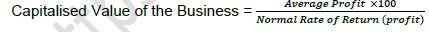Question 9. What is meant by Capitalisation of Super Profit?

In this method, goodwill is calculated by capitalising super profit at the normal rate of return. Thus, as a first step Super Profit is determined on the same basis as is determined under Super Profit Method, which is capitalised at the Normal Rate of Return to determine the value of goodwill.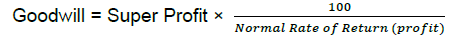Question 10. Why is Goodwill considered as an intangible asset but not a fictitious asset?

Goodwill is a valuable intangible asset like patents, trademarks, copyrights, etc. It is not depreciated like tangible assets but is amortised over its useful life. Intangible Assets prescribes that goodwill should not be recorded in the books of account unless consideration is paid for it.

Fictitious assets are the expenses of a firm such as expenditure on formation of business, debit balance of profit and loss account, deferred revenue expenditure, etc., which are still to be charged against the profits. They all have a debit balance and cannot be considered as assets of the firm.

Question 11. State any two factors affecting the value of Goodwill of a firm.

Goodwill of a firm is affected by all the factors which increase the earning capacity of the firm. These factors are:

(i)   Efficient Management:- If the management is experienced capable and competent, the firm will earn higher profit as compared to other firms. It will, thus, increase the value of goodwill.

(ii) Favourable Location:- If the business is located at a favourable place, resulting in increased customer walk-in and, therefore, sale, the value of goodwill will be higher.

Question 12. How does the factor 'efficiency of management' affect the Goodwill of a firm?

If the management is experienced capable and competent, the firm will earn higher profit as compared to other firms. It will, thus, increase the value of goodwill.

Question 13. How does the factor 'quality of product' affect the Goodwill of a firm?

If a firm enjoys good reputation for the quality of its products, there will be a ready sale and the value of its goodwill, therefore, will be high.

Question 14. How does location affect the Goodwill of a business?

If the business is located at a favourable place, resulting in increased customer walk-in and, therefore, sale, the value of goodwill will be higher.

Question 15. How does the market situation affect the value of Goodwill of a firm?

If a firm is in a business wherein demand for the products dealt in is higher than the supply, it will lead to lower capital requirement and higher profit. It will, thus, increase the value of its goodwill.

Question 16. How does the nature of business affect the value of Goodwill of a firm?

It the business of a firm is of the nature where the products dealt in are in high demand although not short in supply, the profit will be higher. It will, thus, increase the value of its goodwill.

Question 17. Name any four factors which affect the Goodwill of a partnership firm.

Below are the factors affecting the goodwill of a partnership firm:-

1.)   Efficient Management

3.)   Market Situation

Question 18. Give the formula for calculation of Goodwill by Capitalisation of Average Profit.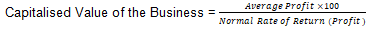Question 19. Give the formula for calculation of Goodwill by 'Capitalisation of Super Profit Method'.Question 20. Enumerate two main steps involved in valuing Goodwill according to Super Profit Method.

Calculating goodwill under this method:-
Step 1. Calculate Super Profit = Actual Average Profit - Normal Profit
Step 2. Goodwill = Super Profit × 100/(Normal Rate of Return)

Question 21. Suraj and Dilip are partners in a firm dealing in stationery items. The firm is well managed and advantage of being cost effective. It buys stationery items at reasonable cost from Dilip's relative manufacturer of stationery items. The firm's sale outlet is situated near a school. As a result, the firm h demand of stationery items and is earning good profits. The firm is donating 10% of its profits to the school for the education of the students of below poverty line. State any two factors affecting the goodwill of the firm. Also, identify any two values which the firm is trying to propagate.

Two factors affecting the value of goodwill of the firm:

(a)   Efficiency of management

(b)   Favourable location

Two Values:

(a)   Sensitivity towards promotion of education among the student of below poverty line.

(b)   Promoting healthy trade practices in the society.

EXERCISE ::-->

Average Profit Method

Question 1:  Goodwill is to be valued at three years' purchase of four years' average profit. Profits for last four years ending on 31st March of the firm were:
2015–Rs.12,000; 2016–Rs.18,000; 2017–Rs.16,000; 2018–Rs.14,000.
Calculate amount of Goodwill.

Goodwill under Average Profit method = Average profit per year × Years Purchase
= 15,000 × 3
= 45,000

Working Note:-

Average profit =  (Total Profit)/(Total Years)

Average profit =  ((12,000+18,000+16,000+14,000)/(4 Years)
Average profit = 15,000

Point of Knowledge:-

Average Profit Method can be calculated by two methods:-
1.) Simple Average Profit Method
2.) Weighted Average Profit Method

Question 2:  The profit for the five years ending on 31st March, are as follows:
Year 2014–Rs 4,00,000 Year 2015–Rs 3,98,000; Year 2016–Rs 4,50,000; Year 2017–Rs 4,45,000; Year 2018–Rs 5,00,000.
Calculate goodwill of the firm on the basis of 4 years' purchase of 5 years' average profit.

Goodwill under Average Profit method = Average profit per year × Years Purchase
= 4,38,600 × 4
= 17,54,400

Working Note:-
Average profit =  (Total Profit)/(Total Years)

Average profit =  ((4,00,000+3,98,000+4,50,000+4,45,000+5,00,000))/(5 Years)
Average profit = 21,93,000/5
Average profit = Rs.4,38,600

Point of Knowledge:-
Steps to calculated Goodwill by Average profit Method:-
Step 1:- Calculate Average profit by the formula = (Total Profit)/(Total Years)

Step 2:- Calculate Goodwill by the formula
= Average Profit × Number of year Purchase (which is given in question)

Question 3:   Calculate value of goodwill on the basis of three years' purchase of average profit of the preceding five years which were as follows: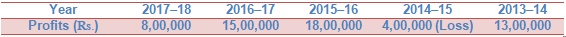Goodwill under Average Profit method = Average profit per year × Years Purchase
= 10,00,000 × 3 years
= 30,00,000

Working Note:-

Average Profit =  (Total Profit)/(Total Years)

Average Profit =  ((8,00,000+15,00,000+18,00,000-4,00,000+13,00,000))/(5 Years)
Average Profit = 50,00,000/(5 Years)
Average Profit = 10,00,000

Point of Knowledge:-

Steps to calculated Goodwill by Average profit Method:-

Step 1:- Calculate Average profit by the formula = (Total Profit)/(Total Years)

Step 2:- Calculate Goodwill by the formula
= Average Profit × Number of year Purchase (which is given in question)

Question 4:  Calculate the value of firm's goodwill on the basis of one and half years' purchase of the average profit of the last three years. The profit for first year was Rs. 1,00,000, profit for the second year was twice the profit of the first year and for the third year profit was one and half times of the profit of the second year.

Goodwill under Average Profit method = Average profit per year × Years Purchase
= Rs. 1,50,000 × 1.5
= Rs. 3,00,000

Working Note:-

Average Profit =  (Total Profit)/(Total Years)

Average Profit =  ((1,00,000)+(1,00,000×2)+(1.00,000×2×1.5))/3Years

Average Profit =  4,50,000/3
Average Profit = 1,50,000

Point of knowledge:-

Steps to calculated Goodwill by Average profit Method:-
Step 1:- Calculate Average profit by the formula = (Total Profit)/(Total Years)

Step 2:- Calculate Goodwill by the formula = Average Profit × Number of year Purchase (which is given in question)

Question 5:   Purav and Purvi are partners in a firm sharing profits and losses in the ratio of 2 : 1. They decide to take Parv into partnership for 1/4th share on 1st April, 2019. For this purpose, goodwill is to be valued at four times the average annual profit of the previous four or five years, whichever is higher. The agreed profits for goodwill purpose of the past five years are: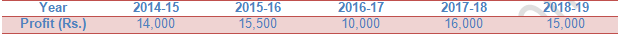Option 1:-

Goodwill under Average Profit method = Average profit per year × Years Purchase

= Rs. 14,100 × 4

= Rs. 56,400

Option 2:-

Goodwill under Average Profit method = Average profit per year × Years Purchase

= Rs. 14,125 × 4

= Rs. 56,500

Goodwill of the firm will be the higher value of the above two options i.e, 56,400 or 56,500. So the goodwill of the firm will be 56,500.

Working Note:-

1.     Calculation of Average profit for Five Years:-

Average Profit =  (Total Profit)/(Total Years)

Average Profit =  (14,000 +15,500 +10,000 +16,000 + 15,000 )/5Years

Average Profit =  70,500/5
Average Profit = 14,100

2.     Calculation of Average profit for Four Years:-

Average Profit =  (Total Profit)/(Total Years)

Average Profit =  (15,500 +10,000 +16,000 + 15,000 )/4Years

Average Profit =  56,500/4

Average Profit = 14,125

Question 6:   Annu, Baby and Chetan are partners in a firm sharing profit and losses equally. They decide to take Deep into partnership from 1st April, 2019 for 1/5th share in the future profit. For this purpose, goodwill is to be valued at 100% of the average annual profit of the previous three or four years, whichever is higher.

The annual profit for the purpose of goodwill for the past four years was:Calculate the value of goodwill.

Option 1:-

Goodwill under Average Profit method = Average profit per year × Years Purchase
= Rs. 3,28,500 × 4
= Rs. 13,14,000

Option 2:-

Goodwill under Average Profit method = Average profit per year × Years Purchase
= Rs. 4,55,100 × 4
= Rs. 18,20,400
Goodwill of the firm will be the higher value of the above two options i.e, 13,14,000 or 18,20,400. So the goodwill of the firm will be 18,20,400.

Working Note:-

Calculation of Average profit for Three Years:-
Average Profit =  (Total Profit)/(Total Years)

Average Profit =  (2,88,000 +1,81,800 +1,87,200 )/3Years

Average Profit =  6,57,000/3
Average Profit = 3,28,500
Calculation of Average profit for Four Years:-

Average Profit =  (Total Profit)/(Total Years)

Average Profit =  (2,88,000 + 1,81,800 +1,87,200 +2,53,200)/4Years

Average Profit =  9,10,200/4

Average Profit = 4,55,100

Point of knowledge:-

Steps to calculated Goodwill by Average profit Method:-
Step 1:- Calculate Average profit by the formula = (Total Profit)/(Total Years)

Step 2:- Calculate Goodwill by the formula = Average Profit × Number of year Purchase (which is given in question)

Question 7:  Divya purchased Jyoti's business with effect from 1st April, 2019. Profits shown by Jyoti's business for the last three financial years were:
2016-17 : Rs. 1,00,000 (including an abnormal gain of Rs. 12, 500).
2017-18 : Rs. 1,25,000 (after charging an abnormal loss of Rs. 25, 000).
2018-19 : Rs. 1,12,500 (excluding Rs. 12,500as insurance premium on firm′s property – now to be insured).
Calculate the value of firm's goodwill on the basis of two year's purchase of the average profit of the last three years.

Average Profit =  (Total Profit)/(Total Years)

Average Profit =  (87,500+1,00,000 +1,50,000 )/3Years

Average Profit =  3,37,500/3
Average Profit = 1,12,500
Goodwill = Average Profits × No. of years of Purchase
= Rs. 1,12,500 × 2
= Rs. 2,25,000

Working Note:-

1. (2016-17) Calculation of Normal Profit = Total Profit – Abnormal gain
= 1,00,000 – 12,500
= 87,500
2. (2017-18) Calculation of Normal Profit = Total Profit – Abnormal gain
= 1,25,000 – 25,000
= 1,00,000
3. (2018-19) Calculation of Normal Profit = Total Profit + Abnormal loss
= 1,12,500 + 12,500
= 1,50,000

Question 8:  Abhay, Babu and Charu are partners sharing profits and losses equally. They agree to admit Daman for equal share of profit. For this purpose, the value of goodwill is to be calculated on the basis of four years' purchase of average profit of last five years. These profits for the year ended 31st March, were:On 1st April, 2018, a car costing Rs. 1,00,000 was purchased and debited to Travelling Expenses Account, on which depreciation is to be charged @ 25%. Interest of Rs. 10,000 on Non-trade Investments is credit to income for the year ended 31st March, 2018 and 2019.
Calculate the value of goodwill after adjusting the above.

Average Profit =  (Total Profit)/(Total Years)

Average Profit =  (1,50,000+3,50,000+5,00,000+7,75,000-6,00,000 )/5Years

Average Profit =  11,75,000/5
Average Profit = 2,35,000
Goodwill = Average Profits × No. of years of Purchase
= Rs. 2,35,000 × 4
= Rs. 9,40,000

Working Note:-

Calculation of Normal Profits for the year 31st March, 2018:-
= Total Profits + Purchase of car wrongly debited - Depreciation on Car - Nontrade Investments
= Rs. 7,10,000 + Rs. 1,00,000 – Rs. 25,000 – Rs.10,000
= Rs. 7,75,000
Calculation of Normal Profits for the year 31st March, 2019:-
= Total Loss + Income from Non - Trade Investments
= 5,90,000 + 10,000
= 6,00,000

Question 9:   Bharat and Bhushan are partners sharing profits in the ratio of 3:2. They decided to admit C as a partner from 1st April, 2018 on the following terms:
Manu will be given 2/5th share of the profit.
Goodwill of the firm is valued at two years' purchase of three years' normal average profit of the firm.
Profits of the previous three years ended 31st March, were:
2018 – Profit Rs 30,000 (after debiting loss of stock by fire Rs 40,000).
2017 – Loss Rs 80,000 (includes voluntary retirement compensation paid Rs 1,10,000).
2016 – Profit Rs 1,10,000 (including a gain (profit) of Rs 30,000 on the sale of fixed assets).
Calculate the value the goodwill.

Goodwill under Average Profit Method = Average Normal Profit per year × Years Purchase
=  (Sum of Three Years Profit)/(Total Number of Years) × Years Purchase
={((1st Years Profit+Abnormal Loss)+(2nd Years loss+Voluntary Retirement Compensation )+(3rd Years Profit-Gain on sale of assets.))/(3 Years)}  ×2 years
= ((30,000+40,000)+(-80,000+1,10,000)+(1,10,000-30,000))/(3 Years) × 2 Years
= (70,000+30,000 +80,000)/(3 Years)  × 2 Years
= 1,80,000/(3 Years) × 2 Year
= 1,20,000

Point of knowledge:-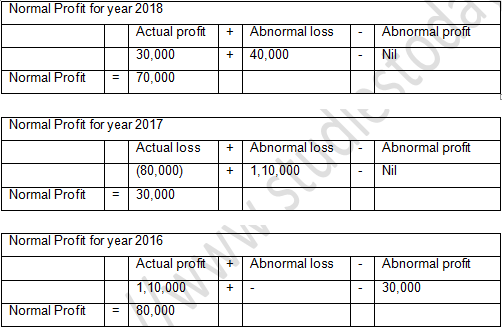Question 10:    Bhaskar and Pillai are partners sharing profits and losses in the ratio of 3:2. They admit Kanika into partnership for 1/4th share in profit. Kanika brings in her share of goodwill in cash. Goodwill for this purpose is to be calculated at two years' purchase of the average normal profit of past three years. Profits of the last three years ended 31st March, were:
2017 - Profit Rs. 50,000 including profit on sale of assets Rs.5, 000.
2018 - Loss Rs.  20,000 including loss by fire Rs. 30, 000.
2019 - Profit Rs.  70,000 (including insurance claim received Rs. 18,000 and interest on investments and Dividend received Rs. 8,000).
Calculate the value of goodwill. Also, calculate goodwill brought in by Kanika.

Average Profit =  (Total Profit)/(Total Years)

Average Profit =  (45,000+10,000 +44,000 )/3Years

Average Profit =  99,000/3
Average Profit = 33,000
Goodwill = Average Profits × No. of years of Purchase
= Rs. 33,000 × 2
= Rs. 66,000
Goodwill brought in by Kanika = Rs. 66,000 × 1/4 = 16,500

Working Note:-

1. (2017) Calculation of Normal Profit = Total Profit – Abnormal gain
= Rs. 50,000 – Rs. 5,000
= Rs. 45,000
2. (2018) Calculation of Normal Profit = Total Loss + Abnormal loss
= Rs. (20,000) + Rs. 30,000
= Rs. 10,000
3. (2019) Calculation of Normal Profit = Total Profit - Abnormal gain
= Rs. 70,000 – Rs. 18,000 – Rs. 8,000
= Rs. 44,000

Question 11:  Sumit purchased Amit's business on 1st April, 2018. Goodwill was decided to be valued at two years' purchase of average normal profit of last four years. The profits for the past four years were: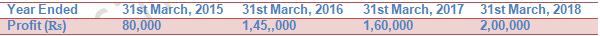Books of Account revealed that:

(i)            Abnormal loss of Rs. 20,000 was debited to Profit and Loss Account for the year ended 31st March, 2015.

(ii)           A fixed asset was sold in the year ended 31st March, 2016 and gain (profit) of Rs. 25,000 was credited to Profit and Loss Account.

(iii)          In the year ended 31st March, 2017 assets of the firm were not insured due to oversight. Insurance premium not paid was Rs. 15,000. Calculate the value of goodwill.

Goodwill under Average Profit Method = Average Normal Profit per year × Years Purchase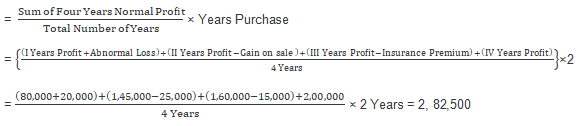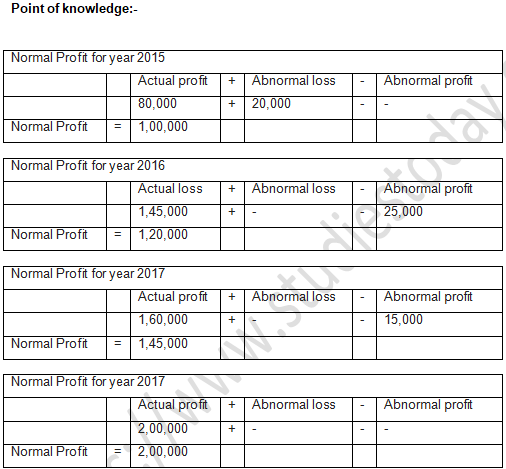Question 12:  Geet and Meet are partners in a firm. They admit Jeet into partnership for equal share. It was agreed that goodwill will be valued at three years' purchase of average profit of last five years. Profits for the last five years were: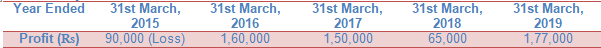Books of Account of the firm revealed that:
(i) The firm had gain (profit) of Rs. 50,000 from sale of machinery sold in the year ended 31st March, 2016. The gain (profit) was credited in Profit and Loss Account.
(ii) There was an abnormal loss of Rs. 20,000 incurred in the year ended 31st March, 2017 because of a machine becoming obsolete in accident.
(iii) Overhauling cost of second hand machinery purchased on 1st July, 2017 amounting to Rs. 1,00,000 was debited to Repairs Account. Depreciation is charged @ 20% p.a. on Written Down Value Method.
Calculate the value of goodwill.

Goodwill under Average Profit Method = Average Normal Profit per year × Years Purchase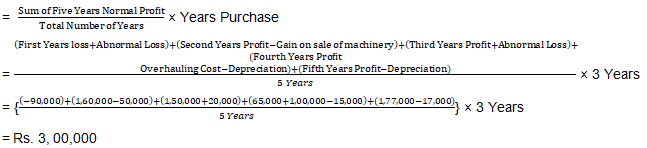Point of Knowledge:-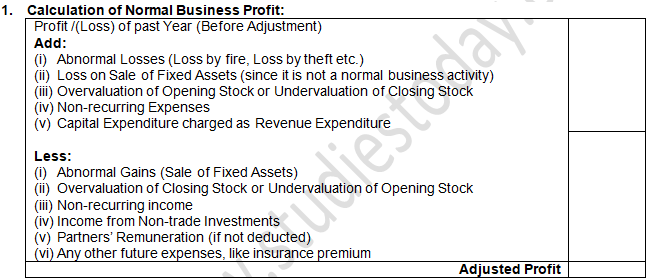Weighted Average Profit Method

Question 13:  Profits of a firm for the year ended 31st March for the last five years were: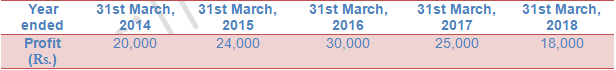Calculate value of goodwill on the basis of three years' purchase of Weighted Average Profit after assigning weights 1, 2, 3, 4 and 5 respectively to the profits for years ended 31st March, 2014, 2015, 2016, 2017 and 2018.

Goodwill under Weighted Average Profit Method
= Weighted Average Profit per Year × Years Purchase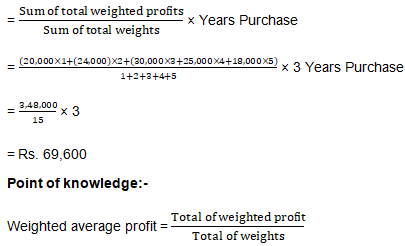Question 14:  A and B are partners sharing profits and losses in the ratio of 5:3. On 1st April, 2019, C is admitted to the partnership for 1/4th share of profits. For this purpose, goodwill is to be valued at two years' purchase of last three years' profits (after allowing partners' remuneration). Profits to be weighted 1:2:3, the greatest weight being given to last year. Net profit before partners' remuneration were: 2016–17: Rs. 2,00,000; 2017–18: Rs. 2,30,000; 2018-19; Rs. 2,50,000. The remuneration of the partners is estimated to be Rs 90,000 p.a. Calculate amount of goodwill.

Goodwill under weighted Average Profit Method

= Weighted Average profit per year × Years Purchase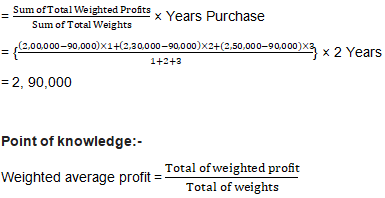Question 15:  Raman and Daman are partners sharing profits in the ratio of 60:40 and for the last four years they have been getting annual salaries of Rs. 50,000 and Rs. 40,000 respectively. The annual accounts have shown the following net profit before charging partners' salaries:

Year ended 31st March, 2017 – Rs. 1,40,000; 2018 – Rs. 1,01,000 and 2019 – Rs. 1,30,000.

On 1st April, 2019, Zeenu is admitted to the partnership for 1/4th share in profit (without any salary). Goodwill is to be valued at four years' purchase of weighted average profit of last three years (after partners′ salaries); Profits to be weighted as 1:2:3, the greatest weight being given to the last year. Calculate the value of Goodwill.Weighted average profit = (Total of weighted profit)/(Total of weights)
= 1,92,000/6
= Rs. 32,000
Goodwill = Weighted Average Profit × No. of Year Purchase
= 32,000 × 4
= Rs. 1, 28,000

Question 16:  Calculate goodwill of a firm on the basis of three years' purchase of the Weighted Average Profit of the last four years. The profits of the last four financial years ended 31st March, were: 2016 – Rs. 25,000; 2017 – Rs. 27,000; 2018 – Rs. 46,900 and 2019 – Rs. 53,810. The weights assigned to each year are: 2016 − 1; 2017 − 2; 2018 − 3; 2019 − 4. You are supplied the following information:

(i)            On 1st April, 2016, a major plant repair was undertaken for Rs. 10,000 which was charged to revenue. The said sum is to be capitalised for goodwill calculation subject to adjustment of depreciation of 10% on Reducing Balance Method.

(ii)           The Closing Stock for the years ended 31st March, 2017 and 2018 were overvalued by Rs.  1,000 and Rs. 2,000 respectively.

(iii)          To cover management cost an annual charge of Rs. 5,000 should be made for the purpose of goodwill valuation.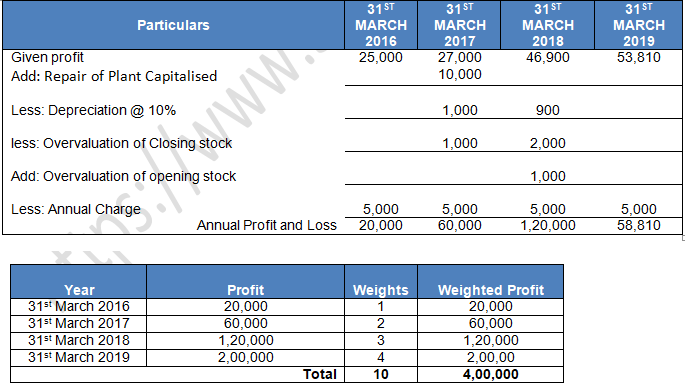Weighted average profit = (Total of weighted profit)/(Total of weights)
= (4,00,000 )/10
= Rs. 40,000
Goodwill = Weighted Average Profit × No. of Year Purchase
= 40,000 × 3
= Rs. 1,12,000

Question 17:  Dinesh and Mahesh are partners sharing profits and losses in the ratio of 3:2. They admit Ramesh into partnership for 1/4th share in profits. Ramesh brings in his share of goodwill in cash. Goodwill for this purpose shall be calculated at two years' purchase of the weighted average normal profit of past three years. Weights being assigned to each year 2017−1; 2018−2 and 2019−3. Profits of the last three years were:

2017 − Profit Rs. 50,000 (including profits on sale of assets Rs. 5, 000.)

2018 − Loss Rs.  20,000 (including loss by fire Rs. 35, 000.)

2019 − Profit Rs.  70,000 (including insurance claim received 18,000 and interest on investments and dividend received Rs. 8, 000.)

Calculate the value of goodwill. Also, calculate the goodwill brought in by Ramesh.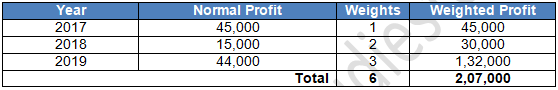Weighted average profit = (Total of weighted profit)/(Total of weights)
= 2,07,000/6
= Rs. 34,500
Goodwill = Weighted Average Profit × No. of Year Purchase
= 34,500 × 2
= Rs. 69,000

Working Note:-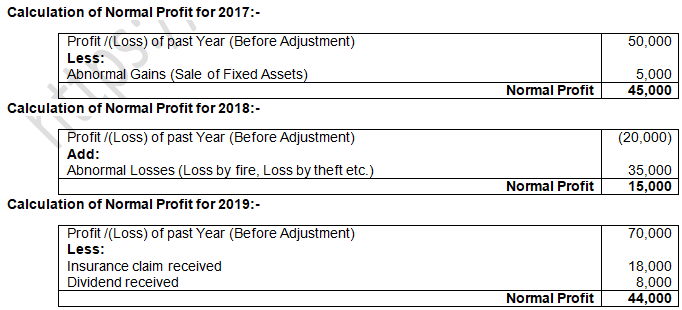Question 18:  Manbir and Nimrat are partners and they admit Anahat into partnership. It was agreed to value goodwill at three years' purchase on Weighted Average Profit Method taking profits of last five years. Weights assigned to each year as 1, 2, 3, 4 and 5 respectively to profits for the year ended 31st March, 2015 to 2019. The profits for these years were: Rs. 70,000, Rs. 1,40,000, Rs. 1,00,000, Rs. 1,60,000 and Rs.1,65,000 respectively.

Scrutiny of books of account revealed following information:

(i)         There was an abnormal loss of Rs. 20,000 in the year ended 31st March, 2015.

(ii)        There was an abnormal gain (profit) of Rs. 30,000 in the year ended 31st March, 2016.

(iii)      Closing Stock as on 31st March, 2018 was overvalued by Rs. 10,000.

Calculate the value of goodwill.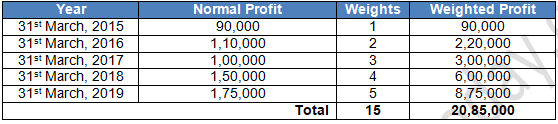Weighted average profit = (Total of weighted profit)/(Total of weights)
= 20,85,000/15
= Rs. 1,39,000
Goodwill = Weighted Average Profit × No. of Year Purchase
= 1,39,000 × 3
= Rs. 4,17,000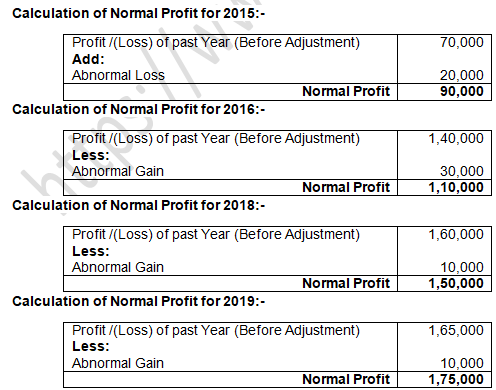Question 19:   Mahesh and Suresh are partners and they admit Naresh into partnership. They agreed to value goodwill at three years' purchase on Weighted Average Profit Method taking profits for the last five years. They assigned weights from 1 to 5 beginning from the earliest year and onwards. The profits for the last five years were as follows: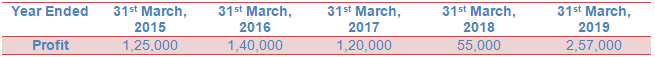Scrutiny of books of account revealed the following:

(i)      A second-hand machine was purchased for Rs. 5,00,000 on 1st July, 2017 and Rs.  1,00,000 were spent to make it operational. Rs. 1,00,000 were wrongly debited to Repairs Account. Machinery is depreciated @ 20% p.a. on Written Down Value Method.

(ii)    Closing Stock as on 31st March, 2018 was undervalued by Rs. 50,000.

(iii)   Remuneration to partners was to be considered as charge against profit and remuneration of Rs.20,000 p.a. for each partner was considered appropriate.

Calculate the value of goodwill.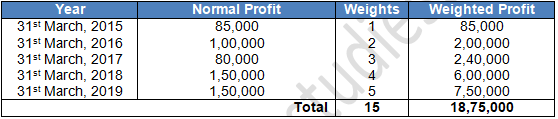Weighted average profit = (Total of weighted profit)/(Total of weights)
= 18,75,000/15
= Rs. 1,25,000

Goodwill = Weighted Average Profit × No. of Year Purchase
= 1,25,000 × 3
= Rs. 3,75,000Question 20:  Calculate the goodwill of a firm on the basis of three years' purchase of the weighted average profit of the last four years. The appropriate weights to be used and profits are:On a scrutiny of the accounts, the following matters are revealed:

(i)            On 1st December, 2017, a major repair was made in respect of the plant incurring Rs. 30,000 which was charged to revenue. The said sum is agreed to be capitalised for goodwill calculation subject to adjustment of depreciation of 10% p.a. on reducing balance method.

(ii)           The closing stock for the year 2016-17 was overvalued by Rs. 12,000.

(iii)          To cover management cost, an annual charge of Rs. 24,000 should be made for the purpose of goodwill valuation.

(iv)         On 1st April, 2016, a machine having a book value of Rs. 10,000 was sold for Rs. 11,000 but the proceeds were wrongly credited to profit and loss account. no effect has been given to rectify the same.

Depreciation is charged on machine @ 10% p.a. on reducing balance method.Weighted average profit = (Total of weighted profit)/(Total of weights)

= 10,41,640/10

= Rs. 1,04,234

Goodwill = Weighted Average Profit × No. of Year Purchase

= 1,04,234 × 3

= Rs. 3,12,702

Working Note:-Super Profit Method

Question 21:   Average profit earned by a firm is Rs. 80,000 which includes undervaluation of stock of Rs. 8,000 on an average basis. The capital invested in the business is Rs. 8,00,000 and the normal rate of return is 8%. Calculate goodwill of the firm on the basis of 7 times the super profit.
Actual Profit = Average Profits + Undervaluation of Stock
= Rs. 80, 000 + Rs. 8, 000
= Rs. 88, 000

Normal Profits = Capital Employed × (Normal Rate of Return)/100
= 8,00,000 × 8/100
= Rs. 64,000

Super Profit = Actual Profit – Normal Profit
= 88,000- 64,000
= 24,000

Goodwill = Super Profit × 4
= 24,000 × 7
= 1,68,000

Working Note:-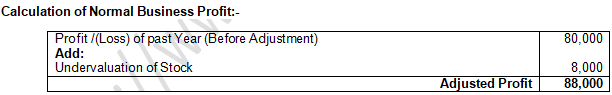Question 22:  Gupta and Bose had a firm in which they had invested Rs. 50,000. On an average, the profits were Rs. 16,000. The normal rate of return in the industry is 15%. Goodwill is to be valued at four years' purchase of profits in excess of profits @ 15% on the money invested. Calculate the value goodwill.

Normal Profit = Capital Employed × (Normal Rate of Return)/100
= 50,000 × 5/100
= Rs. 7,500
Actual Profit = Rs. 16,000 (given)
Super Profit = Actual Profit – Normal Profit
= Rs. 16,000 – Rs. 7,500
= Rs. 8,500
Goodwill = Super Profit × No. of year purchase
= Rs. 8,500 × 4
= Rs. 34,000

Point of Knowledge:-
1. Normal Profit = Capital Employed × (Normal Rate of Return)/100
2. Super Profit = Actual Profit – Normal Profit
3. Goodwill = Super Profit × No. of year purchase

Question 23:  The total capital of the firm of Sakshi, Mehak and Megha is Rs. 1,00,000 and the market rate of interest is 15%. The net profits for the last 3 years were Rs. 30,000; Rs. 36,000 and Rs. 42,000. Goodwill is to be valued at 2 years' purchase of the last 3 years' super profits. Calculate the goodwill of the firm.

Normal Profit = Capital Employed × (Normal Rate of Return)/100
= 1,00,000 × 15/100
= Rs. 15,000
Average Profit = (Total Profit )/(Number of Year)
= (30,000+36,000+42,000)/3
= 1,08,000/3
= Rs. 36,000
Super Profit = Actual Profit – Normal Profit
= Rs. 36,000 – Rs. 15,000
= Rs. 21,000
Goodwill = Super Profit × No. of year purchase
= Rs. 21,000 × 2
= Rs. 42,000

Point of Knowledge:-

Normal Profit = Capital Employed × (Normal Rate of Return)/100
Super Profit = Actual Profit – Normal Profit
Goodwill = Super Profit × No. of year purchase

Question 24:  Rakesh and Ashok earned a profit of Rs. 5,000. They employed capital of Rs. 25,000 in the firm. It is expected that the normal rate of return is 15% of the capital. Calculate amount of goodwill if goodwill is valued at three years' purchase of super profit.

Normal Profit = Capital Employed × (Normal Rate of Return)/100
= 25,000 × 15/100
= Rs. 3,750
Actual Profit = Rs. 5,000 (given)
Super Profit = Actual Profit – Normal Profit
= Rs. 5,000 – Rs. 3,750
= Rs. 1,250
Goodwill = Super Profit × No. of year purchase
= Rs. 1,250 × 3
= Rs. 3,750

Point of Knowledge:-
Normal Profit = Capital Employed × (Normal Rate of Return)/100
Super Profit = Actual Profit – Normal Profit
Goodwill = Super Profit × No. of year purchase

Question 25:   A partnership firm earned net profits during the last three years ended 31st March, as follows: 2016–Rs.17,000; 2017–Rs. 20,000; 2018–Rs. 23,000.
The capital investment in the firm throughout the above-mentioned period has been Rs 80,000. Having regard to the risk involved, 15% is considered to be a fair return on the capital. Calculate value of goodwill on the basis of two years' purchase of average super profit earned during the above-mentioned three years.

Goodwill under Super Profit Method = Super Profit × Years Purchase
= (Average Profit – Normal Profit) × Years Purchase
= (20,000 – 80,000 × 15%) × 2 Years
= (20,000 – 12,000) × 2 Years
= 8,000 × 2 Years
= 16,000

Points of Knowledge:
Average Profit per Year = (Total Sum of Three Years Profit)/(Total Number of Years)
= { (17,000+20,000+23,000)/(3 Years)}
= 20,000

Question 27:  A partnership firm earned net profits during the past three years as follows:Capital investment in the firm throughout the above-mentioned period has been Rs. 4,00,000. Having regard to the risk involved, 15% in considered to be a fair return on the capital. The remuneration of the partners during this period is estimated to be Rs. 1,00,000 p.a.
Calculate value of goodwill on the basis of two years' purchase of average super profit earned during the above-mentioned three years.

Goodwill under Super Profit Method = Super Profit × Years Purchase
= (Average Profit – Partner’s Commission – Normal Profit) × Years Purchase
= (2,00,000 – 1,00,000 – 4,00,000 × 15%) × 2 Years
= (2,00,000 – 1,00,000 – 60,000) × 2 Years
= 40,000 × 2 Years
= 80,000

Points of Knowledge:
Average Profit per Year = (Sum of three years total profit)/(Total Number of years)

= {((1,70,000+2,00,000+2,30,000))/(3 Years)}
= 2,00,000

Question 28:  Ideal Marketing earned an average profit of Rs. 4,00,000 during the last five years. Normal rate of return on capital employed is 10%. Balance Sheet of the firm as at 31st March, 2019 was as follows: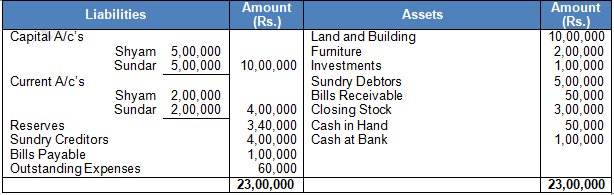Calculate the value of goodwill, if it is valued at three years' purchase of Super Profits.

Normal Profit = Capital Employed × 10/100
= 16,40,000 × 10/100
= 1,64,000
Average Profit = 4,00,000 (Given)
Super Profit = Actual Profit – Normal Profit
= 4,00,000 – 1,64,000
= 2,36,000
Goodwill = Super Profit – Number of Years’ Purchases
= 2,36,000 × 3
= 7,08,000

Working Note:-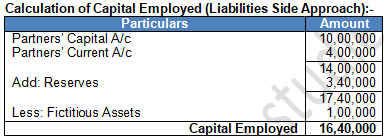Point of Knowledge:-

(a)   Liabilities Side Approach:

Capital Employed = Capital + Reserve – Fictitious Assets – Non-trade Investments

(b)   Assets Side Approach:

Capital Employed = All Assets (except goodwill, fictitious assets and non-trade investments) – Outside Liabilities.

Question 29:  Varuna and Karuna are partners for equal shares. They admit Lata into partnership for 1/4th share. It was agreed to value goodwill of the firm at 4 years' purchase of super profit. Normal rate of return is 15% of the capital employed. Average profit of the firm is Rs. 4,00,000. Balance Sheet of the firm as at 31st March, 2019 was as follows: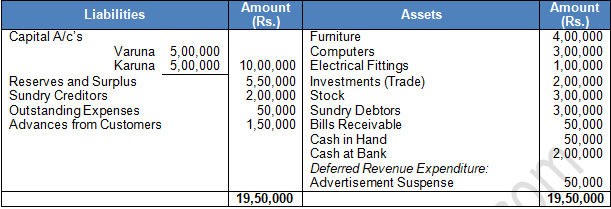Normal Profit = Capital Employed × 15/100
= 15,00,000 × 15/100
= 2,25,000

Average Profit = 4,00,000 (Given)

Super Profit = Actual Profit – Normal Profit
= 4,00,000 – 2,25,000
= 1,75,000
Goodwill = Super Profit – Number of Years’ Purchases
= 1,75,000 × 4
= 7,00,000
Working Note:-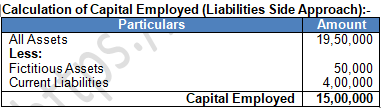Point of Knowledge:-
Liabilities Side Approach:
Capital Employed = Capital + Reserve – Fictitious Assets – Non-trade Investments

Assets Side Approach:
Capital Employed = All Assets (except goodwill, fictitious assets and non-trade investments) – Outside Liabilities.

Question 30:  A business earned an average profit of Rs 8,00,000 during the last few years. The normal rate of profit in the similar type of business is 10%. The total value of assets and liabilities of the business were Rs 22,00,000 and Rs 5,60,000 respectively. Calculate the value of goodwill of the firm by super profit method if it is valued at 21/2 years' purchase of super profits.

Goodwill under Super Profit Method = Super Profit × Year’s Purchase
= (Average Profit – Normal Profits) × Year’s Purchase
= (Average Profit – (Assets – Liabilities) ×10%) × Years Purchase
= (8,00,000 – (22,00,000 – 5,60,000) × 10%) × 2.5 Years Purchase
= (8,00,000 – 16,40,000 × 10%) × 2.5 Years Purchase
= (8,00,000 – 1,64,000) × 2.5 Years Purchase
= Rs. 6,36,000 × 2.5 Years
= Rs. 15,90,000

Question 31:  Capital of the firm of Sharma and Verma is Rs. 2,00,000 and the market rate of interest is 15%. Annual salary to partners is Rs. 12,000 each. The profits for the last three years were Rs. 60,000; Rs. 72,000 and Rs. 84,000. Goodwill is to be valued at 2 years' purchase of last 3 years' average super profit.
Calculate goodwill of the firm.

Goodwill under Super Profit Method = Super Profit × Year’s Purchase
= (Average Profit – Normal Profit) × Year’s Purchase
= (Average Profit – Capital Employed) × % of Normal Rate of Return)×Years Purchase
= (48,000 – 2,00,000 × 15%)×2 years Purchase
= (48,000 – 30,000) × 2 Years
= Rs. 18,000 × 2 Years
= Rs. 36,000

Points of Knowledge :
Average Profit per year = (Sum of Total Normal Profits)/(Total Number of Years)
= { (60,000+72,000+84,000-12,000×2×3×)/(3 Years)}
= 48,000

Question 32:  Supreet and Subham are equal partners. They decide to admit Akriti for 1/3rd share. For the purpose of admission of Akriti, goodwill of the firm is to be valued at four years' purchase of super profit. Average capital employed in the firm is Rs 1,50,000. Normal rate of return may be taken as 15% p.a. Average profit of the firm is Rs 40,000. Calculate value of goodwill.

Goodwill under Super Profit Method = Super Profit × Years Purchase
= (Average Maintainable Profit – Normal Profit) × Years Purchase
= (40,000 – 1,50,000 × 15%) × 4 years Purchase
= (40,000 – 22,500) × 4 years
= 17,500 × 4 years
= 70,000

Question 33:  On 1st April, 2018, an existing firm had assets of Rs 75,000 including cash of Rs 5,000. Its creditors amounted to Rs 5,000 on that date. The firm had a Reserve of Rs 10,000 while Partners' Capital Accounts showed a balance of Rs 60,000. If Normal Rate of Return is 20% and goodwill of the firm is valued at Rs 24,000 at four years' purchase of super profit, find average profit per year of the existing firm.

Goodwill under Super Profit Method:-
Goodwill = Super Profit × Year’s Purchase
Goodwill = (Average Profit – Normal Profit) ×Years Purchase
24,000 = (Average Profit – 14,000) × 4 Years Purchase
(24,000/4) = Average Profit – 14,000
6,000 = Average Profit – 14,000
Average Profit = 14,000 + 6,000
Average Profit = 20,000

Points of Knowledge:

Calculation of Capital Employed:- Assets Approach
Capital Employed = Assets – Creditors
= 75,000 – 5,000
= 70,000
Capital Employed = Capital + Reserve Fund
= 60,000 + 10,000
= 70,000
Normal Rate of Return = 20%
Normal Profit = 70,000 × 20% = 14,000

Question 34:  Average profit earned by a firm is Rs 1,00,000 which includes undervaluation of stock of Rs. 40,000 on an average basis. The capital invested in the business is Rs. 6,30,000 and the normal tare of return is 5%. Calculate goodwill of the firm on the basis of 5 times the super profit.
Goodwill under Super Profit Method = Super Profit × Years Purchase
= Super Profit × Times Purchase
= (Average Profit + Under Valuation of Stock – Normal Profit)×Times Purchase
= (1,00,000 + 40,000 – 6,30,000 × 5%) × 5 Times Purchase
= (1,00,000 + 40,000 – 31,500 ) × 5 Times Purchase
= Rs. 1,08,500 × 5 Times Purchase
= Rs. 5,42,500

Question 35:   The average profit earned by a firm is Rs 7,50,000 which includes overvaluation of stock of Rs 30,000 on an average basis. The capital invested in the business is Rs 4,20,000 and the normal tare of return is 15%. Calculate goodwill of the firm on the basis of 3 time the super profit.

Goodwill under Super Profit Method = Super Profit × Years Purchase
= Super Profit × Times Purchase
= (Average Profit – Over Valuation of Stock – Normal Profit) × Times Purchase
= (7,50,000 – 30,000 – 42,00,000 × 15%) × 5 Times Purchase
= (7,50,000 – 30,000 – 6,30,000) × 5 Times Purchase
= 90,000 × 3 Times Purchase
= Rs. 2,70,000

Question 36:  Ayub and Amit are partners in a firm and they admit Jaspal into partnership w. e. f. 1st April, 2018. They agreed to value goodwill at 3 years' purchase of Super Profit Method for which they decided to average profit of last 5 years. The profit for the last 5 years were: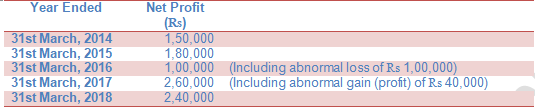The firm has total assets of Rs 20,00,000 and Outside Liabilities of Rs 5,00,000 as on that date. Normal Rate of Return in similar business is 10%.
Calculate value of goodwill.

Value of Goodwill under Super Profit Method
Good Will = Super Profit × Years’ Purchases
= 48,000 × 3Years
= Rs. 1,44,000

Points of Knowledge:

Actual or Average Profit
= { (1,50,000+1,80,000+1,00,000+1,00,000+2,60,000-40,000+2,40,000)/(5 ) }
= 1,98,000
Actual Capital = Assets – Liabilities
= 20,000,00 – 5,00,000
= 15,00,000
Normal Profit = Actual Capital × Rate of Return
= 15,00,000 × 10/100
= 1,50,000
Super Profit = Average Profit – Normal Profit
= (1,98,000 – 1,50,000)
= 48,000

Capitalisation Method

Question 37:  From the following information, calculate value of goodwill of the firm by applying Capitalisation Method: Total Capital of the firm Rs 16,00,000.
Normal rate of return 10%. Profit for the year Rs 2,00,000.
Goodwill under Capitalisation Method
Goodwill = Expected Capital – Actual Capital
Goodwill = Profit for the year × Reverse of Reasonable Rate of Return – Actual Capital
= 2,00,000 × 100/10 – 16,00,000
= 20,00,000 – 16,00,000
= 4,00,000

Question 38:   A business has earned average profit of Rs 1,00,000 during the last few years. Find out the value of goodwill by capitalisation method, given that the assets of the business are Rs 10,00,000 and its external liabilities are Rs 1,80,000. The normal rate of return is 10%.
Actual or Average Profit Rs. 1,00,000
Capitalised value of Average Profit
= 1,00,000 × 100/10
Or Expected Capital = 10,00,000
Actual Capital = Assets – Liabilities
= 10,00,000 – 1,80,000
= Rs. 8,20,000

Value of Goodwill under Capitalisation of Average Profit Method
= Capitalised Value of Average Profit – Actual Capital
= 10,00,000 – 8,20,000
= Rs. 1,80,000

Question 39:  Form the following particulars; calculate value of goodwill of a firm by applying Capitalisation of Average Profit Method:
Profits of last five consecutive years ending 31st March are: 2018–Rs. 54,000; 2017–Rs. 42,000; 2016– Rs.  39,000; 2015– Rs.  67,000 and 2014– Rs.  59,000 Capitalisation rate 20%.
Net assets of the firm Rs 2,00,000.
Value of Goodwill by applying Capitalisation of average profit Method
= Capitalisation of Average Profit or Expected Capital – Actual Capital
= 2,61,000 – 2,00,000
= 61,000

Points of Knowledge:
Average profit per year = (Sum of total Profits)/(Total number of years)
= { (54,000+42,000+39,000+67,000+59,000)/(5 years) }
= 52,200
= Capitalisation of Average Profit or Expected Capital
= 52,200 × 100/20
= 2,61,000
Net Assets = Actual Capital = 2,00,000

Question 40:  A business has earned average profit of Rs 4,00,000 during the last few years and the normal rate of return in similar business is 10%. Find value of goodwill by:
Capitalisation of Super Profit Method.
Super Profit Method if the goodwill is valued at 3 years' purchase of super profits.
Assets of the business were Rs 40,00,000 and its external liabilities Rs 7,20,000.
Actual or Average Profit Rs. 4,00,000
Capitalised Value of Average Profit = 4,00,000 × 100/10
Or Expected capital = 40,00,000
Actual Capital = Assets – Liabilities
= 40,00,000 – 7,20,000
= Rs. 32,80,000
Value of Goodwill under Capitalisation of Average Profit Method
Goodwill = Capitalised value of Average Profit – Actual Capital
= 40,00,000 – 32,80,000 × 10/100
= Rs. 7,20,000
Super Profit = Average Profit – Normal Profit
= 4,00,000 – 32,80,000 × 10/100
= 4,00,000 – 3,28,000
= Rs. 72,000

(1) Value of Goodwill under Capitalisation of Super Profit Method
= Super Profit × Reverse Rate of Return
= 72,000 × 100/10
= 7,20,000

(2) Value of Goodwill under Super Profit Method
= Super profit × Years purchase
= 72,000 × 3
= Rs. 2,16,000

Question 41:  A firm earns profit of Rs. 5,00,000. Normal Rate of Return in a similar type of business is 10%. The value of total assets (excluding goodwill) and total outsiders' liabilities as on the date of goodwill are Rs. 55,00,000 and Rs. 14,00,000 respectively. Calculate value of goodwill according to Capitalisation of Super Profit Method as well as Capitalisation of Average Profit Method.

Total Assets = Assets Excluding Stock + Stock
1,00,000 + 20,000 = 1,20,000
Capital = Assets – Current Liabilities
1,20,000 – 10,000
1,10,000
Goodwill under Super Profit Method = Super Profit × Year’s Purchase
Goodwill under Super Profit Method = (Average Profit – Normal Profit)
60,000 = (Average Profit – 1,10,000 × 8/100) × 4 Years
60,000 = (Average Profit – 8,800) × 4 Years
60,000 + 4 + 8,800 = Average Profit
Average or Actual Profit = 23,800

Question 42:  On 1st April, 2018, a firm had assets of Rs. 1,00,000 excluding stock of Rs. 20,000. The current liabilities were Rs. 10,000 and the balance constituted Partner’s Capital Accounts. If the normal rate of return is 8%, the Goodwill of the firm is valued of Rs. 60,000 at four years’ purchases of super profit, find the actual profits of the firm.

Total Assets = Sundry Assets + Stock
= Rs. 1,00,000 + Rs. 20,000
= Rs. 1,20,000
Current Liabilities = Rs. 10,000
Capital Employed = Total Assets – Current Liabilities
= 1,20,000 – 10,000
= 1,10,000
Normal Profit = Capital Employed × (Normal rate of Return)/100
= Rs. 1,10,000 × 8/100
= Rs. 8,800

Goodwill = Super Profit × No. of Year Purchases
60,000 = (Actual Profit – Normal Profit) × 4
60,000 = (Actual Profit – 8,800)× 4
60,000 = 4× Actual Profit – (8,800 × 4)
60,000 = 4× Actual Profit – 35,200
60,000 + 35,200 = 4× Actual Profit
95,200 = 4× Actual Profit
95,000/4 = Actual Profit
Actual Profit = Rs. 23,800
Capitalisation of Super Profit

Question 43:  Average profit of the firm is Rs 2,00,000. Total assets of the firm are Rs 15,00,000 whereas Partners' Capital is Rs 12,00,000. If normal rate of return in a similar business is 10% of the capital employed, what is the value of goodwill by Capitalisation of Super Profit?
Calculation of Goodwill = Super Profit × 100/(Normal Rate of Return)
= 80,000 × 100/10
= 8,00,000

Working Note:-
Average Profit = 2,00,000 (Given)
Calculation of Super Profit:-
Super Profit = Average Profit – Normal Profit
= Rs. 2,00,000 – Rs. 1,20,000
= Rs. 80,000

Normal Profit = Capital Employed × (Normal Rate of Retur)/100
= Rs. 12,00,000 × 10/100
= Rs. 1,20,000

Point of Knowledge:-

Calculation of Capital Employed:-
Capital Employed = Total Assets – Outside Liabilities
= Rs. 15,00,000 – Rs. 3,00,000
= Rs. 12,00,000

Question 44:  Rajan and Rajani are partners in a firm. Their capitals were Rajan Rs 3,00,000; Rajani Rs 2,00,000. During the year 2017-18, the firm earned a profit of Rs 1,50,000. Calculate the value of goodwill of the firm by capitalisation of super profit assuming that the normal rate of return is 20%.
Goodwill by Capitalisation of Super Profit Method = Super Profit × Reverse Rate of Return
(Actual Profit – Normal Profit) × Reverse of Rate of Return
(Actual Profit – Capital Employed × Normal Rate of Return) × Reverse of Rate of Return
(1,50,000 – 5,00,000 × 20/100 ) × Reverse of Rate of Return
(1,50,000 – 1,00,000 ) × 100/20
= Rs. 50,000 × 100/20
= Rs. 2,50,000

Question 45:  Average profit of GS & Co. is Rs. 50,000 per year. Average capital employed in the business is Rs. 3,00,000. If the normal rate of return of capital employed is 10%, calculate goodwill of the firm by:
Super Profit Method at three years' purchase; and Capitalisation of Super Profit Method.
Goodwill by Super Profit Method at three year’s Purchase:
(Average Profit – Normal Profit) × Years Purchase
(Average Profit – Capital Employed × Normal Rate of Return) × Year’s Purchase
(50,000 – 3,00,000 × 10/100 ) × Year’s Purchase
(50,000 – 30,000) × 3 years Purchase
= 20,000 × 3 Years
= Rs. 60,000

Goodwill by capitalisation of Super Profit Method:
(Average Profit – Normal Profit) × Reverse of Rate of Return
(Average Profit – Capital Employed × Normal Rate of Return) × Reverse of Rate of Return
= (50,000 – 3,00,000 × 10/100 ) × Reverse of Rate of Return
= (50,000 – 30,000) × 100/10
= Rs. 2,00,000

Question 46:  A business has earned average profit of Rs. 8,00,000 during the last few years and the normal rate of return in similar business is 10%. Find value of goodwill by:
Capitalisation of Super Profit Method; and Super Profit Method if the goodwill is valued at 3 years' purchase of super profit.
Assets of the business were Rs. 80,00,000 and its external liabilities Rs. 14,40,000.
Capitalisation of Super Profit = Super Profit × 100/(Normal Rate of Retur)
= 1,44,000 × 100/10
= 14,40,000

Goodwill = Super Profit × No. of Year Purchases
= Rs. 1,44,000 × 3
= Rs. 4,32,000

Working Note:-
Normal Profit = Capital Employed × (Normal Rate of Retur)/100
= Rs. 65,60,000 × 10/100
= Rs. 6,56,000
Average Actual Profit = 8,00,000 (given)
Calculation of Capital Employed = Total Asset – External Liabilities
= Rs. 80,00,000 – Rs. 14,40,000
= Rs. 65,60,000
Super Profit = Average Actual Profit - Normal Profit
= Rs. 8,00,000 – Rs. 6,56,000
= Rs. 1,44,000

Question 47:  Ajeet and Baljeet are partners in a firm. Their capitals are Rs. 9,00,000 and Rs. 6,00,000 respectively. During the year ended 31st March, 2019 the firm earned a profit of Rs. 4,50,000. Assuming that the normal rate of return is 20%, calculate value of goodwill of the firm:
1. By Capitalisation Method; and
2. By Super Profit Method if the goodwill is valued at 2 years' purchase of super profit.
Capitalisation of Super Profit = Super Profit × 100/(Normal Rate of Retur)
= 1,50,000 × 100/20
= 7,50,000
Goodwill = Super Profit × No. of Year Purchases
= Rs. 1,50,000 × 2
= Rs. 3,00,000

Working Note:-
Normal Profit = Capital Employed × (Normal Rate of Retur)/100
= Rs. 15,00,000 × 20/100
= Rs. 3,00,000
Average Actual Profit = 4,50,000 (given)
Calculation of Capital Employed = Total Asset – Current Liabilities
= Rs. 9,00,000 – Rs. 6,00,000
= Rs. 15,00,000
Super Profit = Average Actual Profit - Normal Profit
= Rs. 4,50,000 – Rs. 3,00,000
= Rs. 1,50,000

Question 48:  From the following information, calculate value of goodwill of the firm:
At three years' purchase of Average Profit.
At three years' purchase of Super Profit.
On the basis of Capitalisation of Super Profit.
On the basis of Capitalisation of Average profit.

Information:

Average Capital Employed is Rs 6,00,000.
Net Profit/(Loss) of the firm for the last three years ended are:
31st March, 2108–Rs 2,00,000, 31st March, 2107–Rs 1,80,000, and 31st March, 2106–Rs 1,60,000.
Normal Rate of Return in similar business is 10%.
Remuneration of Rs 1,00,000 to partners is to be taken as charge against profit.
Assets of the firm (excluding goodwill, fictitious assets and not-trade investments) is Rs 7,00,000 whereas Partners' Capital is Rs 6,00,000 and Outside Liabilities Rs 1,00,000.

1. Goodwill at three years purchase of Average profits = Average profit × Years Purchase
=  (Total Profit)/(Total Years)  × Years Purchase
= ((2,00,000+1,80,000+1,60,000-3,00,000)/(3 Years))×3 years
= 80,000 × 3years
= Rs.2,40,000

2. Goodwill by super profit method at three years purchase = Super Profit × Years Purchase
(Average Profit – Normal Profit) × Years Purchase
(Average Profit – Capital Employed × Normal Rate of return) × Years Purchase
=((2,00,000+1,80,000+1,60,000-3,00,000)/3 - 6,00,000 × 10/100) ×Years Purchase
= (80,000 – 60,000) × 3years purchase
= 20,000 × 3Years
= Rs. 60,000

3. Goodwill by Capitalisation Super Profit Method = Super Profit × reverse rate of return
(Average Profit – Normal Profit) × Reverse rate of return
(Average Profit – Capital Employed × Normal Rate of return) × reverse of rate of return
= ((2,00,000+1,80,000+1,60,000-3,00,000)/3 - 6,00,000 × 10/100) × 100/10
= (80,000 – 60,000) × 100/10
= 20,000 × 100/10
= 2,00,000

4. Goodwill by capitalisation of average profit method = Average Profit × reverse rate of return
= ((2,00,000+1,80,000+1,60,000-3,00,000)/3) × 100/10
= Rs. 80,000 × 100/10
= Rs. 8,00,000

Points to Remember in this chapter:-

There are three methods are followed for valuing goodwill:-
1.) Average Profit Method
2.) Super Profit Method
3.) Capitalisation Method
Methods of Valuation of Goodwill:-
I.) Average Profit Method:-
1.) Simple Average Profit Method:- Average Profit × Number of Year’s Purchase
2.) Weighted Average Profit Method:- Weighted Average Profit × Number of Year’s Purchase

II.) Super Profit Method:- Super Profit × Number of year’s Purchase

III.) Capitalisation Method:-
1.) Capitalisation of Average Normal Profit:- Capitalised Value – Net Assets
2.) Capitalisation of Super Profit:- Super Profit × 100 ×100/NRR